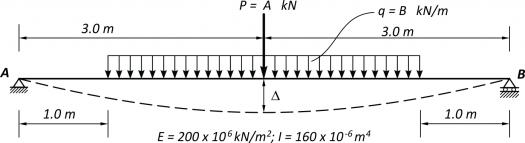# I need help to calculate this beam midspan defflection and combine loadsI need help to calculate this beam midspan defflection using moment area method.. this beam has 2 types of loads, UDL and Point load. please help me.### Re: I need help to calculate this beam midspan defflection...$\delta_{\text{midspan}} = t_{\text{left support}/\text{midspan}}$

$\delta_{\text{midspan}} = \dfrac{1}{EI}(\text{Area}) \, \bar{X}_{\text{left support}}$

$\delta_{\text{midspan}} = \dfrac{1}{EI} \left[ \frac{1}{2}(3C)(2) - \frac{1}{3}(2D)(1.5) \right]$

Substitute the value of C and D and simplify it.

• Mathematics inside the configured delimiters is rendered by MathJax. The default math delimiters are $$...$$ and $...$ for displayed mathematics, and $...$ and $...$ for in-line mathematics.